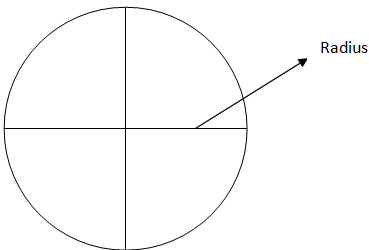QuestionAnswers

# Fill in the blanksAll the radii of the circle are __________.Hint- Basic definition of the circle, also take a look at the figure.All the radii of the circle are equal.
Since, the radii of the circle are a straight line extending from the center of the circle to the circumference. Hence, all the radii of the circle are equal.
Note- Radii of the circle are equal as they are drawn from the center. Also the radius of the circle is half of its diameter.
View NotesWhat are the Domains of the EarthCircumference of a CircleTangent of a CircleEquation of A CircleSecant of a CircleSector of a CircleArea of a CircleParts of CircleAccounting Entries in the Books of the Consignee